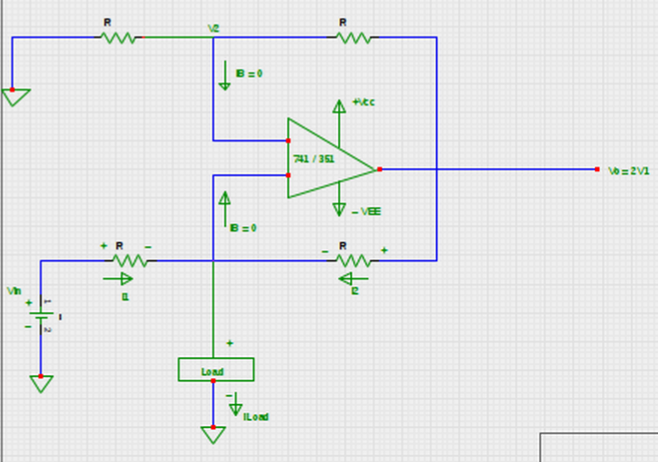# Numerical on Voltage to Current Converter with Grounded Load.

## Determine the values of load current and output voltage respectively for the below schematic of voltage to current converter with grounded load by assuming that the op-amp is nulled at the inception.a. 0.3 mA & 4 V
b. 30 mA & 4 V
c. 300 mA & 2V
d. 30 mA & 2V

Correct Answer : a. 0.3 mA & 4 V

Explanation :

Given data :
From the circuit , the given values are:
Vin= 10 V, R = 30 K & V1 = 2V

We know that load current depends on input voltage resistance R in case of voltage to current converter.

Thus, IL = Vin / R
= 10 V / 30 K
= 0.3 mA

However, the output voltage (Vo) of voltage to current converter is given by,

Vo= 2 V1 =2 x 2 = 4V.